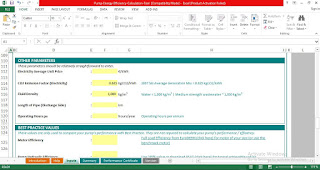Pump Efficiency Calculation Excel Spreadsheets

# Pump Efficiency Calculation Excel SpreadsheetsPump-Efficiency-Calculation-Excel-Spreadsheets

This tool calculates the energy efficiency and energy performance of water (& waste-water) pumping facilities.

To determine pump efficiency you'll need to convert the capacity and head units into horsepower output. Capacity is the total water output, expressed in gallons per minute. Head is the distance from the source water to the pump's output, plus any pressure the pump places on the outlet. Head is expressed in feet.Download Pump-Efficiency-Calculation-Excel-SpreadsheetsDownload Pump-Efficiency-Calculation-Excel-SpreadsheetsDownload Pump-Efficiency-Calculation-Excel-Spreadsheets
Main Inputs:
• Electrical energy consumption (measured or nameplate)
• Run hours
• Discharges pressure (from pressure gauge)
• Flow rate (from flow meter or estimate)
• Average unit price (electricity)
Main Outputs:
• Motor, Pumping (Hydraulic) & System energy efficiencies
• Motor, Pumping & Piping Losses
• Total Energy Consumption & Energy Cost
• CO2 Emissions
• Energy Performance Indicators
• Comparison of Actual Performance v/s Best Practice Performance Certificate